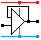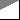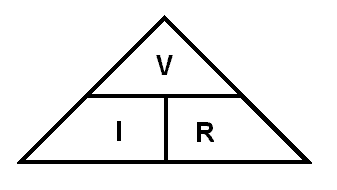Home

rOm
Quest
Glossary

Random
Page
Search
Site
Lush
Sim
Class
Subject
Images

Help
FAQ
Sign
Up
Log
In
 GCSE     Resistors     BS1852     Colour Codes     E24     LDR     Parallel     >PIVR Calculations<     Potentiometer     Puzzles     Series     Thermistor     Timing     Types     Voltage Divider

# Resistors PIVR Calculations

Site for Eduqas/WJEC - Go to the AQA site.

GCSE    ResistorsQuestions 0 to 27   -->  View All

## Definition of Resistance

Resistance ...

• opposes the free flow of current.
• is like a thin water pipe or a narrow road or a narrow doorway opposing the free flow of people.
• is measured in Ohms.
• is related to the current and voltage by Ohm's law.
• if a wire is replaced with a resistor, the current drops.

## Definition of the Ohm

• If the resistance is one Ohm, a potential difference of one Volt will cause a current of one Amp to flow.
• The Ohms tell you how many volts are needed to push one amp through a resistor. For example ...
• 10 Volts are needed to push one amp through a a 10 Ohm resistor.
• 2 Volts are needed to push one amp through a a 2 Ohm resistor.
• 1000000 Volts are needed to push one amp through a one megohm resistor. It would be interesting to watch the sparks fly if you could achieve this in real life!

## Ohm's Law

At a given temperature, the potential difference or Voltage drop (V) across a resistor (R) is proportional to the current (I) flowing through it.

Take Nick Reeder's Ohm's Law Challenge

## Ohm's Law Calculations

R = V / I

• R = resistance in Ohms
• V = Voltage (potential difference) in Volts.
• I = current in Amps.

## Ohm's Law Triangle

This triangle helps some people to remember that

• R = V / I
• V = I R
• I = V / RTo remember this triangle, try this: The Vase on the table Is Red

The ammeter measures the current flowing through the resistor.

The voltmeter measures the voltage across the resistor.

Assume that the meters are ideal and they have no effect on the circuit ...

• No current flows through an ideal voltmeter.
• No voltage is lost across an ideal ammeter.

## Flash Practice

Try a few Ohm's Law calculations ...

## Power Calculations

Resistors come with a power rating. This is the amount of heat that can be dissipated safely. If this rating is exceeded, the resistor will get too hot and could be damaged or even catch fire.

In these calculations ...

• R is the resistance of the resistor measured in Ohms.
• V is the voltage across the resistor measured in Volts. A common mistake is to use some other voltage other than the resistor voltage.
• I is the current through the resistor measured in Amps.
• P is the heat dissipated by the resistor measured in Watts.

P = I V

P = V2 / R

P = I2 R

## Energy Calculations

E   =   P t

Energy is equal to Power (in Watts) x time.

The SI unit of energy is the Joule so one Watt of power for one second would equal one Joule of energy.

When buying electricity, the Joule is an inconveniently small amount so Kilowatt Hours are used instead. This is still Power x Time but Kilowatts and Hours are used instead of Watts and Seconds.

Subject Name     Level     Topic Name     Question Heading     First Name Last Name Class ID     User ID

 CloseEscapeX Q: qNum of last_q     Q ID: Question ID         Score: num correct/num attempts         Date Done

Question Text

image url

Help Text
Debug

• You can attempt a question as many times as you like.
• If you are logged in, your first attempt, each day, is logged.
• To improve your scores, come back on future days, log in and re-do the questions that caused you problems.
• In the grade book, you can delete your answers for a topic before re-doing the questions. Avoid deleting unless you intend re-doing the questions very soon.